# 6-gon

Perimeter of regular hexagon is 113. Calculate its circumradius (radius of circumscribed circle).

R =  18.8

### Step-by-step explanation:

$R=113\mathrm{/}6=18.8$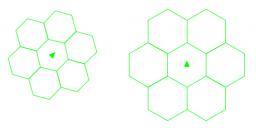We will be pleased if You send us any improvements to this math problem. Thank you!#### You need to know the following knowledge to solve this word math problem:

We encourage you to watch this tutorial video on this math problem:

## Related math problems and questions:

• 6 regular polygonIt is given 6 side regular polygon whose side is 5 cm. Calculate its content area. Compare how many more cm2 (square centimeters) has a circle in which is inscribed the 6-gon.
• n-gon IIWhat is the side length of the regular 5-gon circumscribed circle of radius 11 cm?
• 9-gon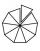Sum of interiol angles of 9-gon is:
• Radius of the circleCalculate the radius of the circle whose length is 107 cm larger than its diameter
• Hexagon areaThe center of the regular hexagon is 21 cm away from its side. Calculate the hexagon side and its area.
• Circle and hexagon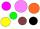Calculate the radius of a circle whose circumference is 8.4 cm longer than the inscribed regular hexagon's circumference.
• Coordinates hexagon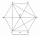The regular hexagon ABCDEF is given. Point A has coordinates [1; 3], and point D has coordinates [4; 7]. Calculate the sum of the coordinates of the center of its described circle.
• Nine-gonCalculate the perimeter of a regular nonagon (9-gon) inscribed in a circle with a radius 13 cm.
• N-gon angles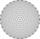What is the sum of interior angles 8-gon? What is the internal angle of a regular convex 8-polygon?
• Regular n-gonWhich regular polygon have a radius of circumscribed circle r = 10 cm and the radius of inscribed circle p = 9.962 cm?
• Hexagon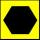Draw a regular hexagon inscribed in a circle with radius r=8 cm. What is its perimeter?
• Quarter circularThe wire that is hooked around the perimeter of quarter-circular arc has length 3π+12. Determine the radius of circle arc.
• Circle from string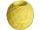Martin has a long 628 mm string. He makes a circle from it. Calculate the radius of the circle.
• Bicycle wheelAfter driving 157 m bicycle wheel rotates 100 times. What is the radius of the wheel in cm?
• Hexagon in circleCalculate the radius of a circle whose length is 10 cm greater than the circumference of a regular hexagon inscribed in this circle.
• The central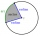The central angle of a sector is 30° and the radius is 15 m. Determine its perimeter.
• Hexagon 5The distance of parallel sides of regular hexagonal is 61 cm. Calculate the length of the radius of the circle described to this hexagon.